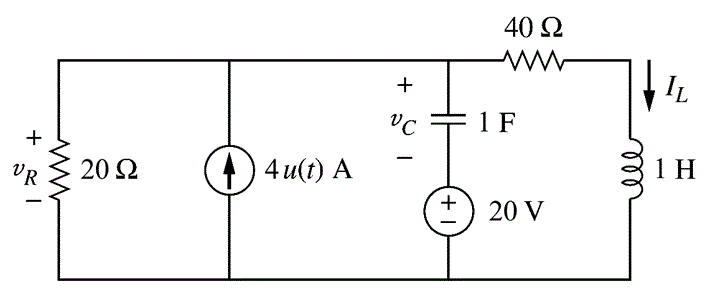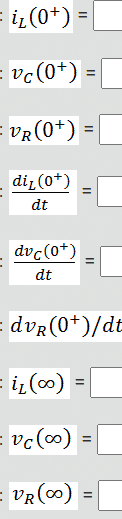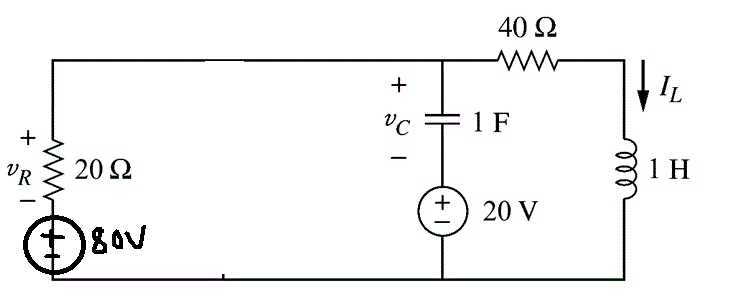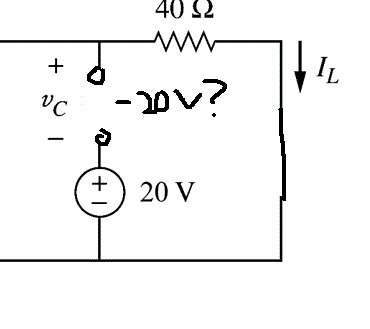# RLC Parallel Circuit

Homework Statement:
Find the desired currents and voltages for the circuit below.
Relevant Equations:
-These are my attempts at doing this question, and I was wondering if I am correct so far.
At t= 0-, i(L) will be 0A, since the capacitor acts as a open circuit.
However, I'm not sure why V(c) at t=0- will be -20V as given by the answers. Won't it be 0V?

Moving on, since current in inductor and voltage in capacitor does not change immediately, the values for t=0+ will be the same as t=0-. (Again, I'm not sure why it is -20V for the capacitor, could someone explain to me?)

I'm not sure how to proceed, but if it was me, I will probably transform the circuit? to:Taking KVL on the left loop,
-80+20i1+ Vc+20=0 --(1)
Taking KVL on right loop,
-20-Vc+40iL+1/L (integerate voltage) -- (2)

I also know Vc = 1/C (integerate i1-iL)

Now the thing is am I making this more complicated than I should? or is this correct so far?
Thanks

cnh1995
Homework Helper
Gold Member
I'm not sure why V(c) at t=0- will be -20V
The voltage source was connected in the circuit for a sufficiently long time. So the circuit was in steady state before the current source was switched at t=0.

You are right about the inductor current and capacitor voltage at t=0+.
Based on this conclusion, what can you say about VR at t=0+?

The voltage source was connected in the circuit for a sufficiently long time. So the circuit was in steady state before the current source was switched at t=0.

You are right about the inductor current and capacitor voltage at t=0+.
Based on this conclusion, what can you say about VR at t=0+?Regarding the voltage, what I do know (from theory is that)it will be a open circuit, but I'm still unclear why it is -20V in this case.

As for the voltage ofthe resistor, won't i need to calculate what is the current running through it using the analysis I listed above? Or am I missing something?

cnh1995
Homework Helper
Gold Member
Regarding the voltage, what I do know (from theory is that)it will be a open circuit, but I'm still unclear why it is -20V in this case.
What is the potential difference across the capacitor at t=0-? You know there is no current through the capacitor. There is no current source in the circuit yet.
Apply KVL and compare it with the reference polarity.
Also, why have you replaced the inductor with a short circuit in your previous post?

cnh1995
Homework Helper
Gold Member
but if it was me, I will probably transform the circuit? to:
That would work if you want to find all of the asked quantities except VR and dVR/dt.
For finding VR and dVR/dt, you need to leave R the way it is, since it behaves differently when clubbed together with the transformed voltage source.

•etotheipi
Also, why have you replaced the inductor with a short circuit in your previous post?
whoops, that was a mistake.

That would work if you want to find all of the asked quantities except VR and dVR/dt.
For finding VR and dVR/dt, you need to leave R the way it is, since it behaves differently when clubbed together with the transformed voltage source.
so if I'm proceeding with the normal circuit, I would need to apply the usual nodal analysis, right?

cnh1995
Homework Helper
Gold Member
so if I'm proceeding with the normal circuit, I would need
Nodal analysis and differential equations would help to find the full response of the circuit. But you are particularly asked about the initial and final values of the response. So you can focus only on initial and final values separately in your analysis.

Initial values first:
You are right about the inductor current and capacitor voltage at t=0+.
When the current source is introduced in the circuit at t=0, the inductor current and capacitor voltage don't change at t=0+. But there is an additional current source in the circuit at t=0+.
Where will this current go at t=0+ ?

Nodal analysis and differential equations would help to find the full response of the circuit. But you are particularly asked about the initial and final values of the response. So you can focus only on initial and final values separately in your analysis.

Initial values first:
You are right about the inductor current and capacitor voltage at t=0+.
When the current source is introduced in the circuit at t=0, the inductor current and capacitor voltage don't change at t=0+. But there is an additional current source in the circuit at t=0+.
Where will this current go at t=0+ ?
can I assume it goes to the resistor only?
Hence voltage of resistor at t=0+ = 80V?
However, the answers given to me stated that it is 0V :/

cnh1995
Homework Helper
Gold Member
Hence voltage of resistor at t=0+ = 80V?
No.
Hint: Voltage across the resistor is the sum of capacitor voltage and 20V source voltage.
You already know the capacitor voltage at t=0- and t=0+.
What does that tell you about the voltage across the resistor at t=0+?

Keep in mind the initial conditions of L and C that you already stated correctly. You need to use them to verify if your assumptions are correct.

No.
Hint: Voltage across the resistor is the sum of capacitor voltage and 20V source voltage.
You already know the capacitor voltage at t=0- and t=0+.
What does that tell you about the voltage across the resistor at t=0+?

Keep in mind the initial conditions of L and C that you already stated correctly. You need to use them to verify if your assumptions are correct.
Ah okay, since voltage across the resistor is the sum of capacitor voltage and 20V source voltage, and voltage at t=0+ of capacitor =-20V, -20+20=0V

As for di/dt at t=0+, I understand that v=L(di/dt). However in this case, will the voltage across the inductor be 0? (even though there is a 40 ohm resistor across the voltages of capacitor and 20V) and the inductor. I would assume so since the answer for di/dt across inductor turns out to be 0.

Also, thinking about dv/dt across capacitor and resistor,the answer turns out to be 4.
For capacitor, i=C(dv/dt). The question is, why will the current going through the capacitor be 4 in this case? Won't some of the current be distributed to the 20 ohm resistor?

cnh1995
Homework Helper
Gold Member
Won't some of the current be distributed to the 20 ohm resistor?
Not at t=0+.
since voltage across the resistor is the sum of capacitor voltage and 20V source voltage, and voltage at t=0+ of capacitor =-20V, -20+20=0V